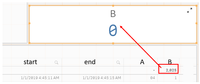# New to Qlik Sense

Discussion board where members can get started with Qlik Sense.

cancel
Showing results for
Did you mean:
HighlightedContributor II

## Count events if interval between 2 dates is less than x seconds

Spoiler
Hi all,

I need to count the events for which the interval between start and end date is less than 40 seconds.
Start and end are datetime values dd/mm/yyyy hh:mm:ss.

Since I'm very new to Qlik Sense I tried to do it step by step.
1st step - calculating the interval between the 2 dates: interval(End- Start, 'ss') as A
2nd step - trying an IF calculation: if(num#(A) < 40, 1, 0) as B

I've tried several ways to count the events based on these 2 steps with no success.
sum(B) returns 0
count(if(num#(A)<40, Event)) also returns 0

Thank you.
Tags (3)
Labels (2)

• ### Interval Dates

5 Replies
HighlightedSpecialist III

## Re: Count events if interval between 2 dates is less than x seconds

you just need to add :

`` if(Time(end-start,'hh:mm:ss')>'00:00:40',1,0) as B,``
Regards,
Taoufiq ZARRA

"Please LIKE posts and "Accept as Solution" if the provided solution is helpful "
HighlightedContributor II

## Re: Count events if interval between 2 dates is less than x seconds

Hi,

I used this formula in a table chart object and it works fine. also, I have the correct value at the total line.

I want to add a KPI object to display the same value. How can I create a KPI based on a calculated measure?

I want the total value of B (shown in the red rectangle) as a KPI by itself.HighlightedSpecialist III

## Re: Count events if interval between 2 dates is less than x seconds

if I understand correctly

=sum(B)

?

Regards,
Taoufiq ZARRA

"Please LIKE posts and "Accept as Solution" if the provided solution is helpful "
HighlightedContributor II

## Re: Count events if interval between 2 dates is less than x seconds

Hi,

I think it should be something as simple as that but I get 0.

HighlightedSpecialist III

## Re: Count events if interval between 2 dates is less than x seconds

can you share your qlik file or a sample data to look

Regards,
Taoufiq ZARRA

"Please LIKE posts and "Accept as Solution" if the provided solution is helpful "